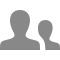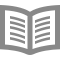## Acerca de este Curso

18,133 vistas recientes

#### 100 % en línea

Comienza de inmediato y aprende a tu propio ritmo.

#### Fechas límite flexibles

Restablece las fechas límite en función de tus horarios.

#### Aprox. 33 horas para completar

Sugerido: 11 hours/week...

#### Inglés (English)

Subtítulos: Inglés (English)

#### 100 % en línea

Comienza de inmediato y aprende a tu propio ritmo.

#### Fechas límite flexibles

Restablece las fechas límite en función de tus horarios.

#### Aprox. 33 horas para completar

Sugerido: 11 hours/week...

#### Inglés (English)

Subtítulos: Inglés (English)

### Gregory PlettInstructor principal

Professor
Electrical and Computer Engineering8,169 alumnos5 cursos

### ofrecido porSemana
1

## Semana 1

5 horas para completar

## The importance of a good SOC estimator

5 horas para completar
8 videos (Total 120 minutos), 13 lecturas, 7 cuestionarios
8 videos
3.1.2: What is the importance of a good SOC estimator?8m
3.1.3: How do we define SOC carefully?16m
3.1.4: What are some approaches to estimating battery cell SOC?26m
3.1.5: Understanding uncertainty via mean and covariance17m
3.1.6: Understanding joint uncertainty of two unknown quantities15m
3.1.7: Understanding time-varying uncertain quantities22m
3.1.8: Summary of "The importance of a good SOC estimator" and next steps3m
13 lecturas
Notes for lesson 3.1.11m
Course Resources5m
How to Use Discussion Forums5m
Earn a Course Certificate5m
Notes for lesson 3.1.21m
Notes for lesson 3.1.31m
Notes for lesson 3.1.41m
Introducing a new element to the course!10m
Notes for lesson 3.1.51m
Notes for lesson 3.1.61m
Notes for lesson 3.1.71m
Notes for lesson 3.1.81m
7 ejercicios de práctica
Practice quiz for lesson 3.1.210m
Practice quiz for lesson 3.1.310m
Practice quiz for lesson 3.1.410m
Practice quiz for lesson 3.1.515m
Practice quiz for lesson 3.1.610m
Practice quiz for lesson 3.1.76m
Quiz for week 140m
Semana
2

## Semana 2

3 horas para completar

## Introducing the linear Kalman filter as a state estimator

3 horas para completar
6 videos (Total 97 minutos), 6 lecturas, 6 cuestionarios
6 videos
3.2.2: The Kalman-filter gain factor23m
3.2.3: Summarizing the six steps of generic probabilistic inference9m
3.2.4: Deriving the three Kalman-filter prediction steps21m
3.2.5: Deriving the three Kalman-filter correction steps16m
3.2.6: Summary of "Introducing the linear KF as a state estimator" and next steps2m
6 lecturas
Notes for lesson 3.2.11m
Notes for lesson 3.2.21m
Notes for lesson 3.2.31m
Notes for lesson 3.2.41m
Notes for lesson 3.2.51m
Notes for lesson 3.2.61m
6 ejercicios de práctica
Practice quiz for lesson 3.2.112m
Practice quiz for lesson 3.2.210m
Practice quiz for lesson 3.2.310m
Practice quiz for lesson 3.2.410m
Practice quiz for lesson 3.2.510m
Quiz for week 230m
Semana
3

## Semana 3

4 horas para completar

## Coming to understand the linear Kalman filter

4 horas para completar
7 videos (Total 86 minutos), 7 lecturas, 7 cuestionarios
7 videos
3.3.2: Introducing Octave code to generate correlated random numbers15m
3.3.3: Introducing Octave code to implement KF for linearized cell model10m
3.3.4: How do we improve numeric robustness of Kalman filter?10m
3.3.5: Can we automatically detect bad measurements with a Kalman filter?14m
3.3.6: How do I initialize and tune a Kalman filter?12m
3.3.7: Summary of "Coming to understand the linear KF" and next steps2m
7 lecturas
Notes for lesson 3.3.11m
Notes for lesson 3.3.21m
Notes for lesson 3.3.31m
Notes for lesson 3.3.41m
Notes for lesson 3.3.51m
Notes for lesson 3.3.61m
Notes for lesson 3.3.71m
7 ejercicios de práctica
Practice quiz for lesson 3.3.110m
Practice quiz for lesson 3.3.210m
Practice quiz for lesson 3.3.310m
Practice quiz for lesson 3.3.410m
Practice quiz for lesson 3.3.510m
Practice quiz for lesson 3.3.610m
Quiz for week 330m
Semana
4

## Semana 4

4 horas para completar

## Cell SOC estimation using an extended Kalman filter

4 horas para completar
8 videos (Total 101 minutos), 8 lecturas, 7 cuestionarios
8 videos
3.4.2: Deriving the three extended-Kalman-filter prediction steps15m
3.4.3: Deriving the three extended-Kalman-filter correction steps6m
3.4.4: Introducing a simple EKF example, with Octave code15m
3.4.5: Preparing to implement EKF on an ECM20m
3.4.6: Introducing Octave code to initialize and control EKF for SOC estimation13m
3.4.7: Introducing Octave code to update EKF for SOC estimation16m
3.4.8: Summary of "Cell SOC estimation using an EKF" and next steps2m
8 lecturas
Notes for lesson 3.4.11m
Notes for lesson 3.4.21m
Notes for lesson 3.4.31m
Notes for lesson 3.4.41m
Notes for lesson 3.4.51m
Notes for lesson 3.4.61m
Notes for lesson 3.4.71m
Notes for lesson 3.4.81m
7 ejercicios de práctica
Practice quiz for lesson 3.4.110m
Practice quiz for lesson 3.4.210m
Practice quiz for lesson 3.4.310m
Practice quiz for lesson 3.4.410m
Practice quiz for lesson 3.4.510m
Practice quiz for lesson 3.4.710m
Quiz for week 430m

## Acerca de Programa especializado Algorithms for Battery Management Systems

In this specialization, you will learn the major functions that must be performed by a battery management system, how lithium-ion battery cells work and how to model their behaviors mathematically, and how to write algorithms (computer methods) to estimate state-of-charge, state-of-health, remaining energy, and available power, and how to balance cells in a battery pack....## Preguntas Frecuentes

• Una vez que te inscribes para obtener un Certificado, tendrás acceso a todos los videos, cuestionarios y tareas de programación (si corresponde). Las tareas calificadas por compañeros solo pueden enviarse y revisarse una vez que haya comenzado tu sesión. Si eliges explorar el curso sin comprarlo, es posible que no puedas acceder a determinadas tareas.

• Cuando te inscribes en un curso, obtienes acceso a todos los cursos que forman parte del Programa especializado y te darán un Certificado cuando completes el trabajo. Se añadirá tu Certificado electrónico a la página Logros. Desde allí, puedes imprimir tu Certificado o añadirlo a tu perfil de LinkedIn. Si solo quieres leer y visualizar el contenido del curso, puedes auditar el curso sin costo.

¿Tienes más preguntas? Visita el Centro de Ayuda al Alumno.